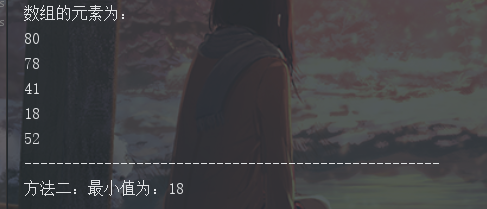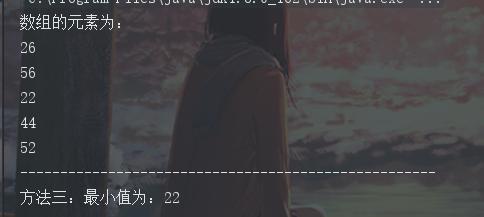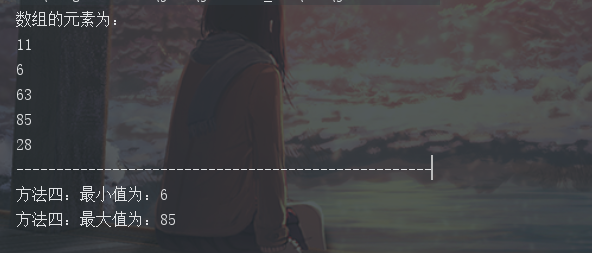• Java输出数组最小值的几种方法 要求： 首先创建一个长度是5的数组 然后给数组的每一位赋予随机整数 找出最小（大）的一个值出来 最小值： 方法一： 该方法来自HOW2J：...
Java输出数组最小值的几种方法
要求：

首先创建一个长度是5的数组
然后给数组的每一位赋予随机整数
找出最小（大）的一个值出来

最小值：
方法一：
该方法来自HOW2J：https://how2j.cn/k/array/array-create/280.html
        int array[] = new int;
System.out.println("数组的元素为：");
for (int i=0;i<array.length;i++){
//为数组元素赋予随机数
array[i] = (int) (Math.random()*100);
System.out.println(array[i]);
}
System.out.println("----------------------------------------------------");
int target = 100;
for(int j=0;j<array.length;j++){
int value = array[j];
System.out.println("value:"+value);
if (value<target){
target = value;
System.out.println("target:"+target);
}
}
System.out.println("方法一，最小值为："+target);

解析：
HOW2J站长说一般找最小值都会先给一个很大的值：
array[i] = (int) (Math.random()*100);

这句代码生成的最大值就是100，无论如何都不会超过100，所以直接定义int target = 100;。然后对数组进行遍历，每遍历一个元素都会赋值给value，然后和target这个最大值进行比较，如果比target小，就把value赋值给target，依次循环判断。
运行结果：
数组的元素为：
53
29
3
50
47
----------------------------------------------------
value:53
target:53
value:29
target:29
value:3
target:3
value:50
value:47
方法一，最小值为：3


方法二：
        int array[] = new int;
System.out.println("数组的元素为：");
for (int i=0;i<array.length;i++){
array[i] = (int) (Math.random()*100);
System.out.println(array[i]);
}
System.out.println("----------------------------------------------------");
int min = array;
for(int i=1;i<array.length;i++)
{
if(min>array[i]){
min=array[i];
}

}
System.out.println("方法二：最小值为："+min);
}方法三：
        int array[] = new int;
System.out.println("数组的元素为：");
for (int i=0;i<array.length;i++){
array[i] = (int) (Math.random()*100);
System.out.println(array[i]);
}
System.out.println("----------------------------------------------------");
//对数组进行排序处理
Arrays.sort(array);
System.out.println("方法三：最小值为："+array);
}方法四：
通过 Collections 类的 Collections.max() 和 Collections.min() 方法来查找数组中的最大和最小值：
        Integer array[] = new Integer;
System.out.println("数组的元素为：");
for (int i=0;i<array.length;i++){
array[i] = (int) (Math.random()*100);
System.out.println(array[i]);
}
System.out.println("----------------------------------------------------");
//通过 Collections 类的 Collections.max() 和 Collections.min() 方法来查找数组中的最大和最小值：
int min = (int)Collections.min(Arrays.asList(array));
int max = (int) Collections.max(Arrays.asList(array));
System.out.println("方法四：最小值为："+min);
System.out.println("方法四：最大值为："+max);展开全文• 把一个数组最开始的若干个元素搬到数组的末尾，我们称之... NOTE：给出的所有元素都大于0，若数组大小为0，请返回0。 方法 直接遍历，找到最小值。 从前往后找,同时从后往前找 二分查找。 import java.util...

把一个数组最开始的若干个元素搬到数组的末尾，我们称之为数组的旋转。 输入一个非减排序的数组的一个旋转，输出旋转数组的最小元素。 例如数组{3,4,5,1,2}为{1,2,3,4,5}的一个旋转，该数组的最小值为1。 NOTE：给出的所有元素都大于0，若数组大小为0，请返回0。

方法

直接遍历，找到最小值。

从前往后找,同时从后往前找

二分查找。

import java.util.ArrayList;
public class Solution {
public int minNumberInRotateArray(int [] array) {

if (array.length == 0) {
return 0;
}

if(array.length==1){
return array;
}

for (int i = 0; i < array.length; i++) {
if (array[i] > array[i + 1]) {
return array[i + 1];
}
}
return 0;

}
}

import java.util.ArrayList;
public class Solution {
public int minNumberInRotateArray(int [] array) {

if (array.length == 0) {
return 0;
}

if(array.length==1){
return array;
}

for (int i = 0; i < array.length; i++) {

//从前往后找
if (array[i] > array[i + 1]) {
return array[i + 1];
}

//从后往前找
if (array[array.length - 1 - i] < array[array.length - 2 - i]) {
return array[array.length - 1 - i];
}
}
return 0;

}
}

import java.util.ArrayList;
public class Solution {
public int minNumberInRotateArray(int [] array) {

if (array.length == 0) {
return 0;
}

if(array.length==1){
return array;
}

int first = 0;
int last = array.length - 1;

while (first < last) {
int mid =  first + (last - first)  / 2;
//中间元素大于最后元素,最小值在右区间
if (array[mid] > array[last]) {
first = mid + 1;
//中间元素小于最后元素,最小值在左区间
} else if (array[mid] < array[last]) {
last = mid;
//相等无法确定区间,但是可以把后指针前移
} else {
last--;
}
}
return array[first];

}
}



展开全文算法
• 例如数组{3,4,5,1,2}为{1,2,3,4,5}的一个旋转，该数组最小值为1。 NOTE：给出的所有元素都大于0，若数组大小为0，请返回0。 //二分法查找定要注意 while(condition) 条件的填写，如果condition无法终止程序，...
把一个数组最开始的若干个元素搬到数组的末尾，我们称之为数组的旋转。 输入一个非递减排序的数组的一个旋转，输出旋转数组的最小元素。 例如数组{3,4,5,1,2}为{1,2,3,4,5}的一个旋转，该数组的最小值为1。 NOTE：给出的所有元素都大于0，若数组大小为0，请返回0。

//二分法查找定要注意 while(condition) 条件的填写，如果condition无法终止程序，则通过里面if 进行终止。
package jianzhiOffer;
import java.util.Scanner;
public class minNumberInRotateArray {
public static void main(String[] args) {
Scanner in = new Scanner(System.in);
String[] str = in.nextLine().trim().split("\\s+");
int[] array = new int[str.length];
for(int i=0;i<str.length;i++)
array[i]=Integer.valueOf(str[i]);
int mid=0,start=0,end=array.length-1;
while(start<end){
mid = (start+end)/2;
if(end-start==1) {
mid =end;
break;
}
if(array[start]==array[end] && array[end]==array[mid]) {
MinInorder(array,start,end);
break;}
if(array[mid]>=array[start])
start=mid;

else if(array[mid]<=array[end])
end =mid;
}
System.out.println(array[mid]);
}

private static void MinInorder(int[] array, int start, int end) {
// TODO Auto-generated method stub
int min=0;
for(int i = start;i<=end;i++)
min = Math.min(min, array[i]);
System.out.print(min);

}
}
展开全文• 题目描述 把一个数组最开始的若干个元素搬到数组... NOTE：给出的所有元素都大于0，若数组大小为0，请返回0。 解题思路：采取栈存取最小值，当数组值小于栈，则push到栈中，最终栈的pop就是最小值 剑指offer的代...
题目描述

把一个数组最开始的若干个元素搬到数组的末尾，我们称之为数组的旋转。 输入一个非减排序的数组的一个旋转，输出旋转数组的最小元素。 例如数组{3,4,5,1,2}为{1,2,3,4,5}的一个旋转，该数组的最小值为1。 NOTE：给出的所有元素都大于0，若数组大小为0，请返回0。

解题思路：采取栈存取最小值，当数组值小于栈，则push到栈中，最终栈的pop就是最小值

剑指offer的代码：

import java.util.ArrayList;
import java.util.Stack;
public class Solution {
public int minNumberInRotateArray(int [] array) {
Stack<Integer> stack = new Stack<Integer>();
stack.push(array);
for(int i=0;i<array.length;i++){
if(array[i]<stack.peek())
stack.push(array[i]);
}
return stack.peek();
}
}

本机测试的代码：
package pratice712;
import java.util.ArrayList;
import java.util.Stack;
public class RotateMinnum712 {
public static int minNumberInRotateArray(int [] array) {
Stack<Integer> stack = new Stack<Integer>();
stack.push(array);
for(int i=0;i<array.length;i++){
if(array[i]<stack.peek())
stack.push(array[i]);
}
return stack.peek();
}
public static void main(String[] args) {
int array[] = {3,4,5,1,2};
int min = minNumberInRotateArray(array);
System.out.print("min: "+min);
}
}

扫码关注一起随时随地学习！！！就在洋葱攻城狮，更多精彩，等你来！！展开全文• NOTE：给出的所有元素都大于0，若数组大小为0，请返回0。 解： 我们把旋转数组看成一个环形数组，首尾相连，只不过缺失了首尾的连接而已。那么原数组的头在“环形数组”中就是那个最小值，而且它的尾就是最大值，...数据结构 算法
• 例如数组{3,4,5,1,2}为{1,2,3,4,5}的一个旋转，该数组最小值为1。 NOTE：给出的所有元素都大于0，若数组大小为0，请返回0。 第一遍读题没想明白…看了下题解后发现自己想复杂了… 首先用for循环写了个答案…没想到...
• 循环有序数组最小值局部最小值位置定义局部最小的概念。arr长度为1时，arr是局部最小。arr的长度为N(N>1)时，如果arrpublic class Solution { public int getLessIndex(int[] arr) { if (arr == null || arr...数据结构
• 例如数组{3,4,5,1,2}为{1,2,3,4,5}的一个旋转，该数组最小值为1。 NOTE：给出的所有元素都大于0，若数组大小为0，请返回0。 一、Python： 1.1 直接使用min函数解题，但不足以拿到offer # -*- coding:ut...Python Scala
• //从后向前依次比较，遇到大的数停止，返回；否则最小的是第一个位置的元素 for(int i=numbers.length-1;i>0;i--){ if(numbers[i-1]>numbers[i])return numbers[i]; } return numbers; } }java
• 分析到这里，就知道我们其实关心的是以某个数字结尾时的子数组最小值之和，可以用一个一维数组 dp，其中 dp[i] 表示以数字 A[i] 结尾的所有子数组最小值之和，将 dp 初始化为 A，结果 res 也初始化为 A。...
• Java数组获取最大和最小值 方法： 通过使用Collection类的Collection.max()和Collection.min()方法来查找数组中的最大和最小值 代码： import java.util.Arrays; import java.util.Collections; public class ...
• DK8特性：java最大值（整数数组）： int[] A = {6,7,8,2}; int maxVal = Arrays.stream(A).max()....JDK8特性：java最小值（整数数组）： int[] A = {6,4,5}; int minVal = Arrays.stream(A).min().getAsInt(); ...
• 如果输入整数为2，则调用打印数组最小值printMin的方法，将该数组的最小值打印出来 PrintMax0和printmin 0需要自己定义 package 小测试; import java.util.Scanner; public class 第二题 { public static void ...
• Java获取数组最大值和最小值 声明2个变量，取数组任意两个值进行赋值 遍历数组，分别与这两值比较，比当前最大值大时，把它赋值给最大值，比当前最小值小时，把它赋值给最小值。 public class GetMaxMin ...算法 编程语言
• 例如数组{3,4,5,1,2}为{1,2,3,4,5}的一个旋转，该数组最小值为1。 NOTE：给出的所有元素都大于0，若数组大小为0，请返回0。分析: 考察二分查找。旋转之后的数组实际上可以划分成两个有序的子数组：前面子数组的...
• 例如数组{3,4,5,1,2}为{1,2,3,4,5}的一个旋转，该数组最小值为1。 NOTE：给出的所有元素都大于0，若数组大小为0，请返回0。可以借助二分查找法的思想。/** * @author 16026 * */ public class Min {二分查找
• 获取数组最大值和最小值 废话少说，直接上代码，说白了就是基础自己学，方法可以参考 import java.util.Arrays; public class Test_获取最大值和最小值 { public static void main(String[] args) { //定义一个...
• Java语言用函数实现返回数组中最大值最小值和平均值，请给我代码 Java语言用函数实现返回数组中最大值最小值和平均值，请给我代码 Java语言用函数实现返回数组中最大值最小值和平均值，请给我代码开发语言
• 数组中存放10个随机数，输出数组并查找该数组最小值
• 设置两个临时变量t1，t2，然后遍历数组，t1始终保存较大值，t2保存较小值，遍历完毕，就能得到一个最大值t1，最小值t2。 (2)求java数组相邻值大于某个数，比如10，打印出新的数组，这个东西是做数据分析的，把...
• 比较二维数组最小值，组成一个新数组返回。(实现核心算法，不需要使用IO) 输入：intArr = {{5,6,1,16},{7,3,9}} 输出：intArrs ={1,3} import java.util.Arrays; /** * 比较二维数组最小值，组成一个新数组...
• import java.util.Arrays;... // 求出数组最大值 最小值 int[] arr = arr(20);//用一个数组接收返回的值 System.out.println(Arrays.toString(arr));//打印一下所创建的数组 System.out.println(max(arr
• 功能需求:遍历数组,并求出数组中元素的最大元素,最小元素,及其相应的索引等问题,要求用方法完成. 思路:分别创建不同的方法,然后再调用方法. 代码展示: public class Array{ public static void main(String[] ...遍历数组
• ## Java获取数组最大值

万次阅读 多人点赞 2018-07-31 09:31:45
简述 方法其实有很多种的。我先放一些，之后有再遇到对应代码再放上来。 最简单的当然是一个个找进行...import java.util.Arrays; public static int MAX(int[] arr) { Arrays.sort(arr); return arr[arr.len...最大值
• 获得最大值及最大值索引 public static int[] getMaxIndex(int[] arr) { if(arr==null||arr.length==0){...//如果数组为空 或者是长度为0 就返回null } int maxIndex=0;//假设第一个元素为最大值 那么下标设为0 ...
• 数组最小值  public String mymin(int[] arr){    int min=arr;  for(int i=0;i;i++){  if(arr[i]){  min=arr[i];  }  }  return "数组的最小值为："+min;  }  //4.交换方法  public ...二分查找
• 文章目录题目描述思路及解答 题目描述 ...例如数组{3, 4, 5, 1, 2}为{1, 2, 3, 4, 5}的一个旋转，该数组最小值为1。 思路及解答 import java.util.Scanner; import java.io.*; public class...
• 例如，数组 [3,4,5,1,2] 为 [1,2,3,4,5] 的一个旋转，该数组最小值为1。 来源：力扣（LeetCode） 链接：https://leetcode-cn.com/problems/xuan-zhuan-shu-zu-de-zui-xiao-shu-zi-lcof 著作权归领扣网络所有。商业...二分法 算法 leetcode golang...

# java返回数组最小值java 订阅